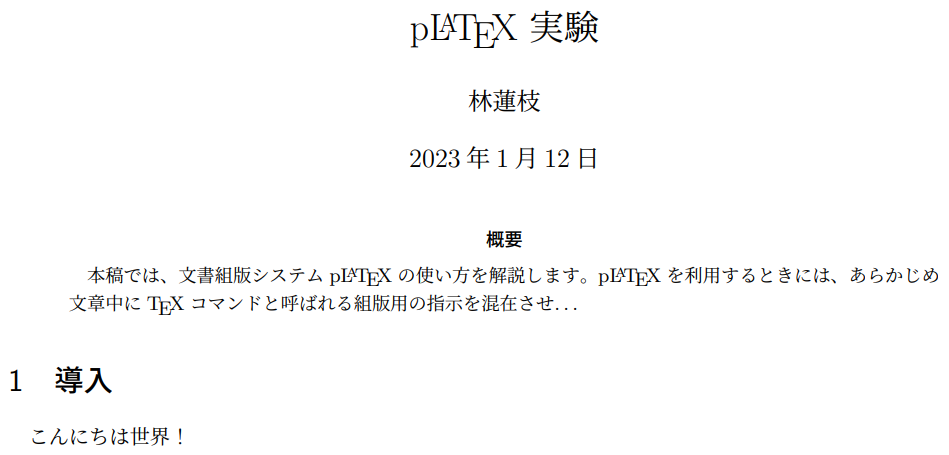Yes, Overleaf can compile Japanese documents that require pTeX. Here's how:

1. A pTeX document does not need special commands for Japanese text (kanji, hiragana and katakana). Here's a simple example (using the jsarticle document class):
\documentclass{jsarticle}

\bibliographystyle{jplain}
\title{p\LaTeX\ 実験}
\author{林蓮枝}

\begin{document}

\maketitle

\begin{abstract}
本稿では、文書組版システムp\LaTeX{}の使い方を解説します。p\LaTeX{}を利用するときには、あらかじめ文章中に\TeX{}コマンドと呼ばれる組版用の指示を混在させ\ldots
\end{abstract}

\section{導入}
こんにちは世界！

\end{document}

2. If you are uploading a .tex file from elsewhere, make sure it is saved in UTF-8 encoding.
3. In the Overleaf editor, click on the Overleaf menu button above the file list panel.
4. Set the "Compiler" option to "LaTeX".
5. Add a blank file to your project, and save it as latexmkrc.
6. Add the following line to your latexmkrc file:
7. $latex = 'platex';$bibtex = 'pbibtex';
$dvipdf = 'dvipdfmx %O -o %D %S';  Use the link below the following code listing to open an Overleaf project which creates a suitable latexmkrc file and typesets the LaTeX code. main.tex \documentclass{jsarticle} \bibliographystyle{jplain} \title{p\LaTeX\ 実験} \author{林蓮枝} \begin{document} \maketitle \begin{abstract} 本稿では、文書組版システムp\LaTeX{}の使い方を解説します。p\LaTeX{}を利用するときには、あらかじめ文章中に\TeX{}コマンドと呼ばれる組版用の指示を混在させ\ldots \end{abstract} \section{導入} こんにちは世界！ \end{document}  latexmkrc $latex = 'platex';
$bibtex = 'pbibtex';$dvipdf = 'dvipdfmx %O -o %D %S';


This example produces the following output: